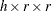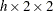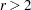The FREQ Procedure
 ODS Graphics

PROC FREQ assigns a name to each graph that it creates with ODS Graphics. You can use these names to refer to the graphs. Table 3.15 lists the names of the graphs that PROC FREQ generates together with their descriptions and the options that are required to produce the graphs.

To request graphics with PROC FREQ, you must first enable ODS Graphics by specifying the ODS GRAPHICS ON statement. See Chapter 21, Statistical Graphics Using ODS (SAS/STAT 9.22 User's Guide), for more information. When you have enabled ODS Graphics, you can request specific plots with the PLOTS= option in the TABLES statement. If you do not specify the PLOTS= option but have enabled ODS Graphics, then PROC FREQ produces all plots that are associated with the analyses that you request.

Table 3.15 ODS Graphics Produced by PROC FREQ

ODS Graph Name

Plot Description

TABLES Statement Option

CumFreqPlot

Cumulative frequency plot

One-way table request

DeviationPlot

Deviation plot

CHISQ and a one-way table request

FreqPlot

Frequency plot

Any table request

KappaPlot

Kappa plot

AGREE (table)

ORPlot

Odds ratio plot

MEASURES or RELRISK (table)

RelRiskPlot

Relative risk plot

MEASURES or RELRISK (table)

RiskDiffPlot

Risk difference plot

RISKDIFF (table)

WtKappaPlot

Weighted kappa plot

AGREE (table,)Previous Page | Next Page | Top of Page